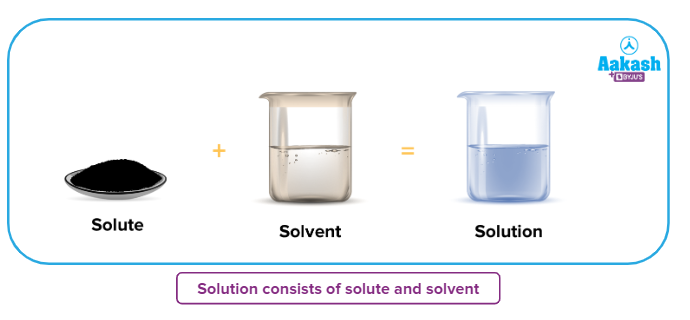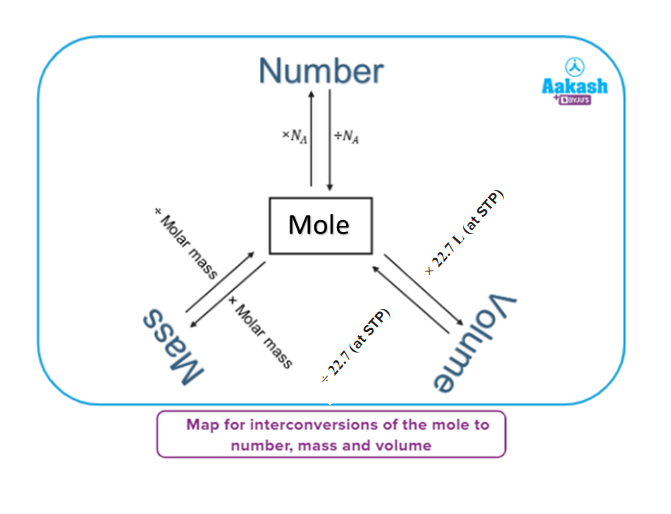• Call Now

1800-102-2727•

# Mole concept: Mole and its needs-Definitions, Practice problems

Have you seen a Rs. 100 note? Probably yes. How about Rs. 1000? Probably yes. Most of us have seen the common denominations of Indian currency ranging from Rs 10 to Rs 2000. All of these denominations help in the transaction of money.

Similarly, while dealing with chemical reactions, one has to take the help of numerical denominations to specify quantities of matter getting consumed or produced. Atoms being such a small unit, it's much harder to deal with a number of atoms as a unit of measurement. In order to overcome this problem, Italian scientist Amedeo Avogadro is credited with coming up with the concept of “mole” to identify the number of particles present.

He was the first to realize that the volume of a gas (strictly, of an ideal gas) is proportional to the number of atoms or molecules. (We will study Avagadro’s Law in States of Matter).

To put it into context, what is the value of one mole? Let me give you some (mind-blowing) observations!!! Are you ready?

1. If you had exactly one mole of sheets of paper, you could make one million equal stacks from sea level on the earth that would pass the sun.
2. If you had a mole of pennies, you could give out enough money to everyone in the world so that they could spend a million dollars every hour, day and night, for the rest of their lives.
3. If you wanted to use trial and error to find the combination for an e-mail password, if it contained exactly six alphanumeric characters, it would take you up to 636 different tries, which is approximately 1028, which is over 17,000 moles.

So the natural question would be, “Why should we study mole?”

The mole concept is a convenient method of expressing the amount of a substance. Any measurement can be broken down into two parts – the numerical magnitude and the magnitude’s units. For example, when a ball’s mass is measured to be 2 kilograms, the magnitude is ‘2’ and the unit is ‘kilogram’. When dealing with particles at an atomic (or molecular) level, even one gram of a pure element is known to contain a huge number of atoms. This is where the mole concept is widely used. It primarily focuses on the unit known as a ‘mole’, which is a count of a very large number of particles.

• Mole
• Mathematical forms of mole
• Standard temperature and pressure (STP)
• Normal temperature and pressure (NTP)
• Practice problems

## Mole

We know one Dozen=12 entities

Entities can be a pen, pencil, etc, but entities can’t be atoms, ions, molecules, etc)

We know one

$6.022×{10}^{23}$ number is known as Avogadro’s number and is represented by NA.

$6.022×{10}^{23}$ = 1 NA

We can say,

1 mole of H+ion = Collection of $6.022×{10}^{23}{H}^{+}ion$

1 mole of H2 molecules = Collection of

1 mole of H atoms = Collection of

The quantity “mole” can be utilised for either a homogenous or heterogenous mixture. The concept of mole can be utilised to solute or solvent depending upon one’s requirement.A mole is defined as the amount of substance comprising the same number of fundamental entities as the number of atoms present in a pure sample of carbon weighing exactly 12 g or A mole is defined as the amount of a substance that contains exactly 6.0221023 elementary entities of the given substance.

Why did we take Avogadro's number to be $6.022×{10}^{23}$

We know, the mass of one proton =$1.66×{10}^{-24}g$

$⇒\frac{1}{1.66×{10}^{-24}}\approx 6.022×{10}^{23}$

1 amu= $1.66×{10}^{-24}g$

Also remember: 1 amuNA1

## Mathematical forms of mole

1. Mole-to-number relation

1

We know, number of dozens =

Similarly, the number of moles =

1. Mole - weight relation

Number of moles =

The mass of 6.0221023 particles of any substance is termed the molar mass of the substance.

1. Mole-volume relationship

Number of moles = volume of gas (L)Molar volume (L) (at same T & P)

At STP,

The number of moles =

## Standard temperature and pressure (STP)

Temperature =

Pressure = 1 atm

It was experimentally observed that, at STP conditions, one mole of an ideal gas occupies 22.7 L volume.

## Normal temperature and pressure (NTP)

Temperature =

Pressure = 1 atm

It was experimentally observed that, at NTP conditions, one mole of an ideal gas occupies 24.0 L volume.## Practice problems

Q1. Find the total number of Helium (He) atoms present in 2.5 moles of Helium.

a. NA
b. 2NA
c. 2.5NA
d. 3NA

Solution: we know 1 mole = $6.022×{10}^{23}$ entities

The total number of He atoms = 2.5 $6.022×{10}^{23}$ = 2.5NA

Q2. Find the total mass in grams of 3.5 moles of copper.(Molar mass of Cu = 63.5 g mol-1)

a. 222.25 g
b. 250.45 g
c. 234.34 g
d. 342.76 g

Solution: number of moles =

Weight of substance = (3.5 63.5) g = 222.25 g

Q 3. Find the volume at STP for 64 g SO2 gas.

a. 44.8 L
b. 22.4 L
c. 11.2 L
d. 5.6 L

Solution: number of moles of

number of moles =

Volume of SO2 gas = 1 mol 22.4 L=22.4 L

Q4. Find the charge of 54 mg of Al3+ ions.

a. 144.56 C
b. 72.30 C
c. 96.35 C
d. 192.7 C

Solution: number of moles of

The total number of Al3+ ions =

One ion Al3+ contains three positive charges.

We know the magnitude of charge of one proton equals the magnitude of charge of one electron.

Charge of one proton = $1.6×{10}^{-19}C$

Total charge on Al3+=

Q. What is the meaning of gram-atom and gram-molecule?
According to modern practice, gram-atom and gram-molecule are known as moles.

E.g- 1 gram-atom Na+ = 1 mol of Na+

1 gram-molecule H2O = 1 mol of H2O

Q. When is Mole Day celebrated?
$1{N}_{A}=6.022×{10}^{23}$

Mole Day is celebrated on September 23, from 6 AM to 6 PM.

Q. Why do we use mole fraction?
The mole fraction defines the number of single component molecules (or moles) divided by the total number of molecules (or moles) in the mixture. When two reactive components are mixed together, the mole fraction is useful, as the ratio of the two components is understood if the mole fraction of each is known.

Q. Is mole fraction equal to partial pressure?
In a mixture, the partial pressure of each gas is proportional to its mole fraction. The pressure exerted by each gas (its partial pressure) in the gas mixture is independent of the pressure exerted by all other gases present in the gas mixture.

Q. What is the importance of the mole concept?
All chemistry is pervaded by the mole definition. Since most quantitative chemical calculations are focused on the mole, for the study of chemistry, an understanding of the mole is important. Knowledge of how the mole applies to mass, the number of entities, etc.Talk to our expert
Resend OTP Timer =
By submitting up, I agree to receive all the Whatsapp communication on my registered number and Aakash terms and conditions and privacy policy